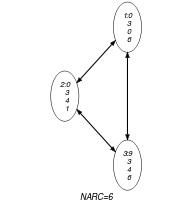## 5.4. all_differ_from_exactly_k_pos

Origin
Constraint

$\mathrm{𝚊𝚕𝚕}_\mathrm{𝚍𝚒𝚏𝚏𝚎𝚛}_\mathrm{𝚏𝚛𝚘𝚖}_\mathrm{𝚎𝚡𝚊𝚌𝚝𝚕𝚢}_𝚔_\mathrm{𝚙𝚘𝚜}\left(𝙺,\mathrm{𝚅𝙴𝙲𝚃𝙾𝚁𝚂}\right)$

Type
 $\mathrm{𝚅𝙴𝙲𝚃𝙾𝚁}$ $\mathrm{𝚌𝚘𝚕𝚕𝚎𝚌𝚝𝚒𝚘𝚗}\left(\mathrm{𝚟𝚊𝚛}-\mathrm{𝚍𝚟𝚊𝚛}\right)$
Arguments
 $𝙺$ $\mathrm{𝚒𝚗𝚝}$ $\mathrm{𝚅𝙴𝙲𝚃𝙾𝚁𝚂}$ $\mathrm{𝚌𝚘𝚕𝚕𝚎𝚌𝚝𝚒𝚘𝚗}\left(\mathrm{𝚟𝚎𝚌}-\mathrm{𝚅𝙴𝙲𝚃𝙾𝚁}\right)$
Restrictions
 $\mathrm{𝚛𝚎𝚚𝚞𝚒𝚛𝚎𝚍}$$\left(\mathrm{𝚅𝙴𝙲𝚃𝙾𝚁},\mathrm{𝚟𝚊𝚛}\right)$ $|\mathrm{𝚅𝙴𝙲𝚃𝙾𝚁}|\ge 1$ $|\mathrm{𝚅𝙴𝙲𝚃𝙾𝚁}|\ge 𝙺$ $𝙺\ge 0$ $\mathrm{𝚛𝚎𝚚𝚞𝚒𝚛𝚎𝚍}$$\left(\mathrm{𝚅𝙴𝙲𝚃𝙾𝚁𝚂},\mathrm{𝚟𝚎𝚌}\right)$ $\mathrm{𝚜𝚊𝚖𝚎}_\mathrm{𝚜𝚒𝚣𝚎}$$\left(\mathrm{𝚅𝙴𝙲𝚃𝙾𝚁𝚂},\mathrm{𝚟𝚎𝚌}\right)$
Purpose

Enforce all pairs of distinct vectors of the $\mathrm{𝚅𝙴𝙲𝚃𝙾𝚁𝚂}$ collection to differ from exactly $𝙺$ positions. Enforce $𝙺=0$ when $|\mathrm{𝚅𝙴𝙲𝚃𝙾𝚁𝚂}|<2$.

Example
$\left(2,〈\mathrm{𝚟𝚎𝚌}-〈0,3,0,6〉,\mathrm{𝚟𝚎𝚌}-〈0,3,4,1〉,\mathrm{𝚟𝚎𝚌}-〈9,3,4,6〉〉\right)$

The $\mathrm{𝚊𝚕𝚕}_\mathrm{𝚍𝚒𝚏𝚏𝚎𝚛}_\mathrm{𝚏𝚛𝚘𝚖}_\mathrm{𝚎𝚡𝚊𝚌𝚝𝚕𝚢}_𝚔_\mathrm{𝚙𝚘𝚜}$ constraint holds since:

• The first and second vectors differ from 2 positions, which is equal to $𝙺=2$.

• The first and third vectors differ from 2 positions, which is equal to $𝙺=2$.

• The second and third vectors differ from 2 positions, which is equal to $𝙺=2$.

Typical
 $𝙺>0$ $𝙺<|\mathrm{𝚅𝙴𝙲𝚃𝙾𝚁}|$ $|\mathrm{𝚅𝙴𝙲𝚃𝙾𝚁𝚂}|>1$
Symmetries
• Items of $\mathrm{𝚅𝙴𝙲𝚃𝙾𝚁𝚂}$ are permutable.

• Items of $\mathrm{𝚅𝙴𝙲𝚃𝙾𝚁𝚂}.\mathrm{𝚟𝚎𝚌}$ are permutable (same permutation used).

Arg. properties

Contractible wrt. $\mathrm{𝚅𝙴𝙲𝚃𝙾𝚁𝚂}$.

implies: $\mathrm{𝚊𝚕𝚕}_\mathrm{𝚍𝚒𝚏𝚏𝚎𝚛}_\mathrm{𝚏𝚛𝚘𝚖}_\mathrm{𝚊𝚝}_\mathrm{𝚕𝚎𝚊𝚜𝚝}_𝚔_\mathrm{𝚙𝚘𝚜}$ ($=𝙺$ replaced by $\ge 𝙺$), $\mathrm{𝚊𝚕𝚕}_\mathrm{𝚍𝚒𝚏𝚏𝚎𝚛}_\mathrm{𝚏𝚛𝚘𝚖}_\mathrm{𝚊𝚝}_\mathrm{𝚖𝚘𝚜𝚝}_𝚔_\mathrm{𝚙𝚘𝚜}$ ($=𝙺$ replaced by $\le 𝙺$).

Keywords
Cond. implications

$\mathrm{𝚊𝚕𝚕}_\mathrm{𝚍𝚒𝚏𝚏𝚎𝚛}_\mathrm{𝚏𝚛𝚘𝚖}_\mathrm{𝚎𝚡𝚊𝚌𝚝𝚕𝚢}_𝚔_\mathrm{𝚙𝚘𝚜}\left(𝙺,\mathrm{𝚅𝙴𝙲𝚃𝙾𝚁𝚂}\right)$

with  $𝙺\le |\mathrm{𝚅𝙴𝙲𝚃𝙾𝚁𝚂}|$

implies $\mathrm{𝚊𝚝𝚕𝚎𝚊𝚜𝚝}_\mathrm{𝚗𝚟𝚎𝚌𝚝𝚘𝚛}$$\left(\mathrm{𝙽𝚅𝙴𝙲},\mathrm{𝚅𝙴𝙲𝚃𝙾𝚁𝚂}\right)$.

Arc input(s)

$\mathrm{𝚅𝙴𝙲𝚃𝙾𝚁𝚂}$

Arc generator
$\mathrm{𝐶𝐿𝐼𝑄𝑈𝐸}$$\left(\ne \right)↦\mathrm{𝚌𝚘𝚕𝚕𝚎𝚌𝚝𝚒𝚘𝚗}\left(\mathrm{𝚟𝚎𝚌𝚝𝚘𝚛𝚜}\mathtt{1},\mathrm{𝚟𝚎𝚌𝚝𝚘𝚛𝚜}\mathtt{2}\right)$

Arc arity
Arc constraint(s)
$\mathrm{𝚍𝚒𝚏𝚏𝚎𝚛}_\mathrm{𝚏𝚛𝚘𝚖}_\mathrm{𝚎𝚡𝚊𝚌𝚝𝚕𝚢}_𝚔_\mathrm{𝚙𝚘𝚜}$$\left(𝙺,\mathrm{𝚟𝚎𝚌𝚝𝚘𝚛𝚜}\mathtt{1}.\mathrm{𝚟𝚎𝚌},\mathrm{𝚟𝚎𝚌𝚝𝚘𝚛𝚜}\mathtt{2}.\mathrm{𝚟𝚎𝚌}\right)$
Graph property(ies)
$\mathrm{𝐍𝐀𝐑𝐂}$$=|\mathrm{𝚅𝙴𝙲𝚃𝙾𝚁𝚂}|*|\mathrm{𝚅𝙴𝙲𝚃𝙾𝚁𝚂}|-|\mathrm{𝚅𝙴𝙲𝚃𝙾𝚁𝚂}|$

Graph class
 $•$$\mathrm{𝙽𝙾}_\mathrm{𝙻𝙾𝙾𝙿}$ $•$$\mathrm{𝚂𝚈𝙼𝙼𝙴𝚃𝚁𝙸𝙲}$

Graph model

The Arc constraint(s) slot uses the $\mathrm{𝚍𝚒𝚏𝚏𝚎𝚛}_\mathrm{𝚏𝚛𝚘𝚖}_\mathrm{𝚎𝚡𝚊𝚌𝚝𝚕𝚢}_𝚔_\mathrm{𝚙𝚘𝚜}$ constraint defined in this catalogue.

Parts (A) and (B) of Figure 5.4.1 respectively show the initial and final graph associated with the Example slot. Since we use the $\mathrm{𝐍𝐀𝐑𝐂}$ graph property, the arcs of the final graph are stressed in bold. The previous constraint holds since exactly $3·\left(3-1\right)=6$ arc constraints hold.

##### Figure 5.4.1. Initial and final graph of the $\mathrm{𝚊𝚕𝚕}_\mathrm{𝚍𝚒𝚏𝚏𝚎𝚛}_\mathrm{𝚏𝚛𝚘𝚖}_\mathrm{𝚎𝚡𝚊𝚌𝚝𝚕𝚢}_𝚔_\mathrm{𝚙𝚘𝚜}$ constraint(a) (b)
Signature

Since we use the $\mathrm{𝐶𝐿𝐼𝑄𝑈𝐸}\left(\ne \right)$ arc generator on the items of the $\mathrm{𝚅𝙴𝙲𝚃𝙾𝚁𝚂}$ collection, the expression $|\mathrm{𝚅𝙴𝙲𝚃𝙾𝚁𝚂}|·|\mathrm{𝚅𝙴𝙲𝚃𝙾𝚁𝚂}|-|\mathrm{𝚅𝙴𝙲𝚃𝙾𝚁𝚂}|$ corresponds to the maximum number of arcs of the final graph. Therefore we can rewrite the graph property $\mathrm{𝐍𝐀𝐑𝐂}$ $=$ $|\mathrm{𝚅𝙴𝙲𝚃𝙾𝚁𝚂}|·|\mathrm{𝚅𝙴𝙲𝚃𝙾𝚁𝚂}|-|\mathrm{𝚅𝙴𝙲𝚃𝙾𝚁𝚂}|$ to $\mathrm{𝐍𝐀𝐑𝐂}$ $\ge$ $|\mathrm{𝚅𝙴𝙲𝚃𝙾𝚁𝚂}|·|\mathrm{𝚅𝙴𝙲𝚃𝙾𝚁𝚂}|-|\mathrm{𝚅𝙴𝙲𝚃𝙾𝚁𝚂}|$. This leads to simplify $\underline{\overline{\mathrm{𝐍𝐀𝐑𝐂}}}$ to $\overline{\mathrm{𝐍𝐀𝐑𝐂}}$.